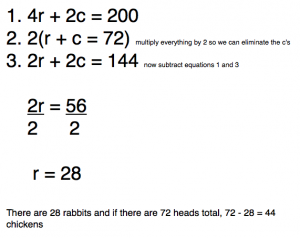# Week 4

This week we continued working on problem solving strategies.  We looked at writing an equation to solve a problem and while that is not my most favorite way to solve problems, it is a strategy that will help students in future years of math.  Can you think of any real world situations where you use equations to solve problems?  If so, we’d love to hear from you!

Just to give you an example of the types of problems we set up with an equation…

• A number plus 5 is 7.  What is that number?  Even though kids can do this problem in their head, we talked about translating word problems into equations and the proper steps to show when solving equations.   x + 5 = 7  When you subtract 5 from both sides, you get x = 3.  We talked about isolating the variable and performing the opposite operation to both sides of the equation.
• The 6th graders took it a step further and looked at systems of equations.  There are several chickens and rabbits in a cage.  There are 72 heads and 200 feet inside the cage.  How many chickens are there?  How many rabbits?  One equation is:  r + c = 72 since there are a total of 72 heads.  Rabbits have 4 feet and chickens have 2 feet so the other equation is 4r + 2c = 200.  This system of equations can be solved by multiplication and elimination.In addition to math problem solving strategies, we went over Lesson 1 from Wordly Wise.  Students should all have Lesson 2 now and in addition to working on the different sections in the packet, students were asked to pick one word from the list and illustrate it for a technology activity we’ll do in the next couple weeks.

The EY coordinators are working hard to prepare for next Friday’s Strategy Seminar.  If you haven’t signed the permission form yet, please do so and have your child return it as soon as possible.  I will make sure to take lots of pictures and post them next week. 🙂

Have a great weekend!